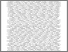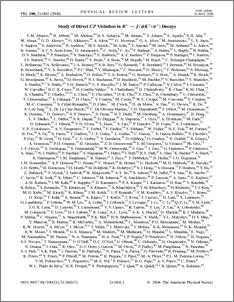# Study of direct CP violation in $B^{\pm} \to J/ψK^{\pm}(π^{\pm})$ decays

Collaboration, D0 and Abazov, V. and Bertram, Iain and Williams, Mark and Borissov, Guennadi and Ratoff, Peter and Fox, Harald (2008) Study of direct CP violation in $B^{\pm} \to J/ψK^{\pm}(π^{\pm})$ decays. Physical review letters, 100 (21). ISSN 1079-7114Preview
PDF
e211802.pdf - Published Version

## Abstract

We present a search for direct CP violation in $B^{\pm} \to J/\psi K^{\pm}(\pi^{\pm})$ decays. The event sample is selected from 2.8 fb$^{-1}$ of $p\bar{p}$ collisions recorded by D0 experiment in Run II of the Fermilab Tevatron Collider. The charge asymmetry $A_{CP}(B^+ \to J/\psi K^+) = +0.0075 \pm 0.0061$(stat.)$\pm 0.0027$(syst.) is obtained using a sample of approximately 40 thousand $B^{\pm} \to J/\psi K^{\pm}$ decays. The achieved precision is of the same level as the expected deviation predicted by some extensions of the standard model. We also measured the charge asymmetry $A_{CP}(B^+ \to J/\psi \pi^+) = -0.09 \pm 0.08$(stat.)$\pm 0.03$(syst.).

Item Type:
Journal Article
Journal or Publication Title:
Physical review letters
© 2008 The American Physical Society
Uncontrolled Keywords:
/dk/atira/pure/researchoutput/libraryofcongress/qc
Subjects:
?? PHYSICSPHYSICS AND ASTRONOMY(ALL)QC PHYSICS ??
Departments:
ID Code:
56737
Deposited By:
Deposited On:
07 Aug 2012 09:04
Refereed?:
Yes
Published?:
Published